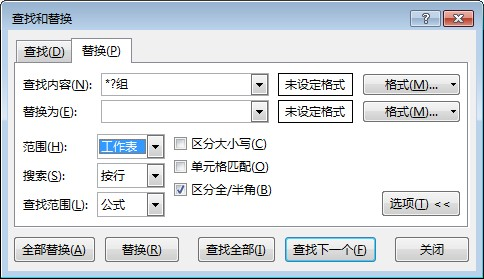﻿ 如何在vba中使用excel的查找替换功能？ - VBA - ExcelOffice【微信公众号：水星Excel】

# 如何在vba中使用excel的查找替换功能？excel内置的查找功能具有很强大的功能，不仅可以在当前工作表中进行查找，还可以在整个工作簿中查找。

```Sub QQ1722187970()
Dim oRng As Range
Dim oWK As Worksheet
'遍历每个工作表
For Each oWK In Excel.Worksheets
'在有数据的单元格查找
With oWK.UsedRange
'找到第一个满足条件的单元格
Set oRng = .Find("*?组")
If Not (oRng Is Nothing) Then
'保存第一个找到的单元格的地址
'开始执行代码
Do
'循环每一个满足条件的单元格
Set oRng = .FindNext(oRng)
'开始执行代码
'直到回到第一个被找到的单元格
End If
End With
Next
MsgBox "操作完成"
End Sub
```

```Sub QQ1722187970()
Application.ScreenUpdating = False
Dim oRng As Range
Dim oWK As Worksheet
Set oDic = CreateObject("Scripting.Dictionary")
For Each oWK In Excel.Worksheets
oDic.RemoveAll
With oWK.UsedRange
Debug.Print oWK.Name
Set oRng = .Find("*?组")
If Not (oRng Is Nothing) Then

Do
Set oRng = .FindNext(oRng)

End If
arrKey = oDic.keys
arrItem = oDic.items
For i = 0 To UBound(arrItem)
If Right(arrItem(i), 1) = "组" And Len(arrItem(i)) > 1 Then
.Range(arrKey(i)).Value = VBA.Replace(arrItem(i), "组", "")
End If
Next i
End With
Next
MsgBox "替换完成"
Application.ScreenUpdating = True
End Sub
```